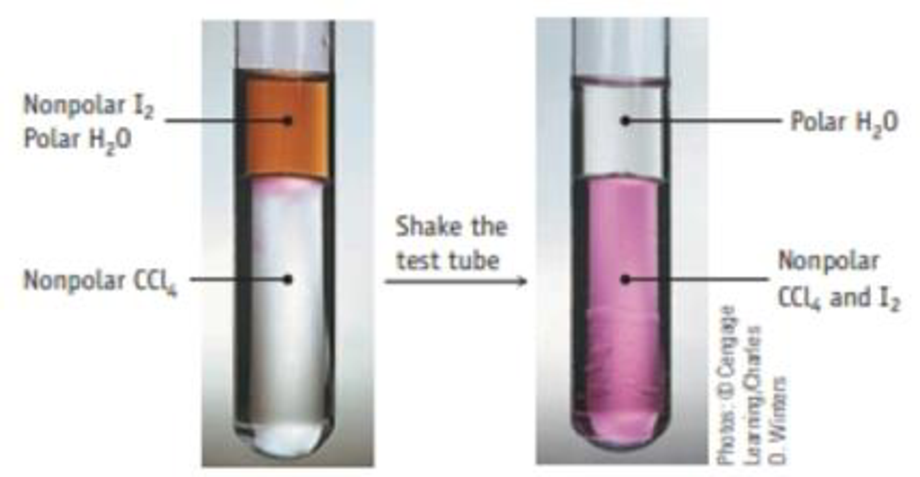Chapter 15, Problem 18PS

Chapter
Section
Textbook Problem

Iodine dissolves in water, but its solubility in a nonpolar solvent such as CCl4 is greater.Extracting iodine (I2) from water with the nonpolar solvent CCl4. I2 is more soluble in CCl4 and, after shaking a mixture of water and CCl4, the I2 has accumulated in the more dense CCl4 layer.The equilibrium constant is 85.0 for the processI2(aq) ⇄ I2(CCl4)You place 0.0340 g of I2 in 100.0 mL of water. After shaking it with 10.0 mL of CCl4, how much I2 remains in the water layer?

Interpretation Introduction

Interpretation: Amount of I2(in gram) remains in water layer in the given reaction have to be calculated.

Concept introduction:

• Equilibrium constant: At equilibrium the ratio of products to reactants has a constant value. And it is represented by the letter K.

For a general reaction, aA+bBcC+dD

The equilibrium constant Kc = [C]c[D]d[A]a[B]b, where a, b, c and d are the stoichiometric coefficients of reactant and product in the reaction. Concentration value for solid substance is 1.

If the value of Kc and the concentration of any of the reactant of a reaction is known then the concentration of product can be determined by multiplying Kc with the concentration of reactant.

• Concentration =AmountofsubstanceVolume
• Amountofsubstance=Concentration×Volume
• Amountofsubstance=MassingramMolarmass
• Massofthesubstance=Amountofthesubstance×molarmass
• ICE (reaction initial concentration equilibrium) table is mainly used to calculate the value of K for a reaction. This table contains the concentration of reactant and product in various stage of reaction.
Explanation

The balanced equation for the given reaction is,

I2(aq)I2(CCl4)

The value of Kc for this reaction is 85.0

Amount of I2 available =  0.0340g253.8g/mol=0.000134mol

The concentration of I2 = 0.000134mol0.1L=0.00134mol/L

By using this concentration ICE table for this reaction can be constructed as follows,

 Reaction                                        I2(aq)                ⇄              I2(CCl4) Initial concentration(mol/L) 0

Still sussing out bartleby?

Check out a sample textbook solution.

See a sample solution

The Solution to Your Study Problems

Bartleby provides explanations to thousands of textbook problems written by our experts, many with advanced degrees!

Get Started